# HC-SR04 Ultrasonic sensor and STM32

Ultrasonic ranging module HC – SR04 provides 2cm – 400cm non-contact measurement function, the ranging accuracy can reach to 3mm.
The modules includes ultrasonic transmitters, receiver and control circuit.
Today in this tutorial we are going to learn How to interface HCSR04 Ultrasonic sensor module with STM32.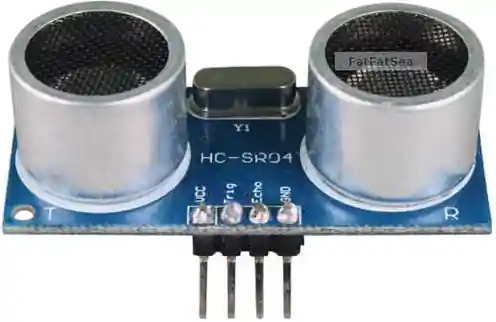hcsr04

## WORKING

Working of hcsr04 is pretty simple and straight.
The module emits an ultrasound at 40 KHz which, after reflecting from obstacle, bounces back to the module.
By using the travel time and the speed of the sound, we can calculate the distance between the sensor and the obstacle.

## HOW TO

According to the datasheet of hc-sr04, the following is required to be done :-

• Keep the Trig pin HIGH for at least 10us
• The Module will now send 8 cycle burst of ultrasound at 40 kHz and detect whether there is a pulse signal back
• IF the signal returns, module will output a HIGH PULSE whose width will be proportional to the range of the object.
• Distance can be calculated by using the following formula :- range = high level time * velocity (340m/s) / 2
• We can also use uS / 58 = Distance in cm or uS / 148 = distance in inch
• It is recommended to wait for at least 60ms before starting the operation again.

#### Method to use

As mentioned above, the module sends a HIGH signal proportional to the distance measured. This signal remains high for few microseconds. To measure the width of the signal in microseconds, I will use the Input Capture to measure this Pulse Width.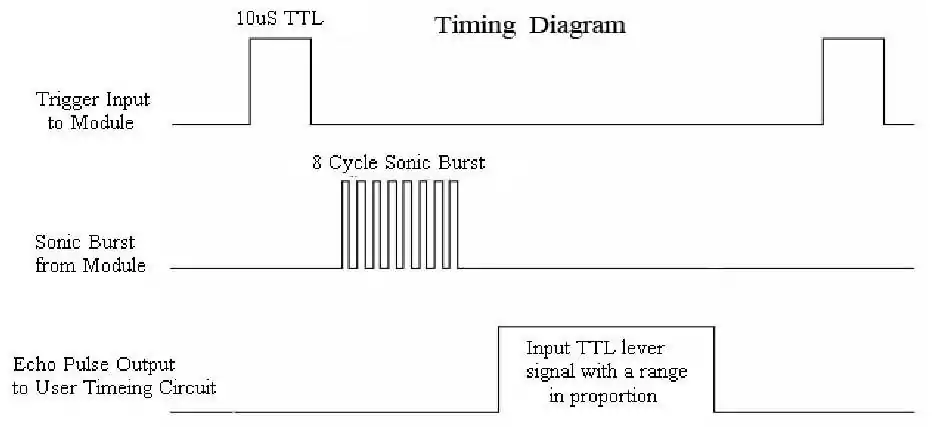hcsr04 timing

## Setup Required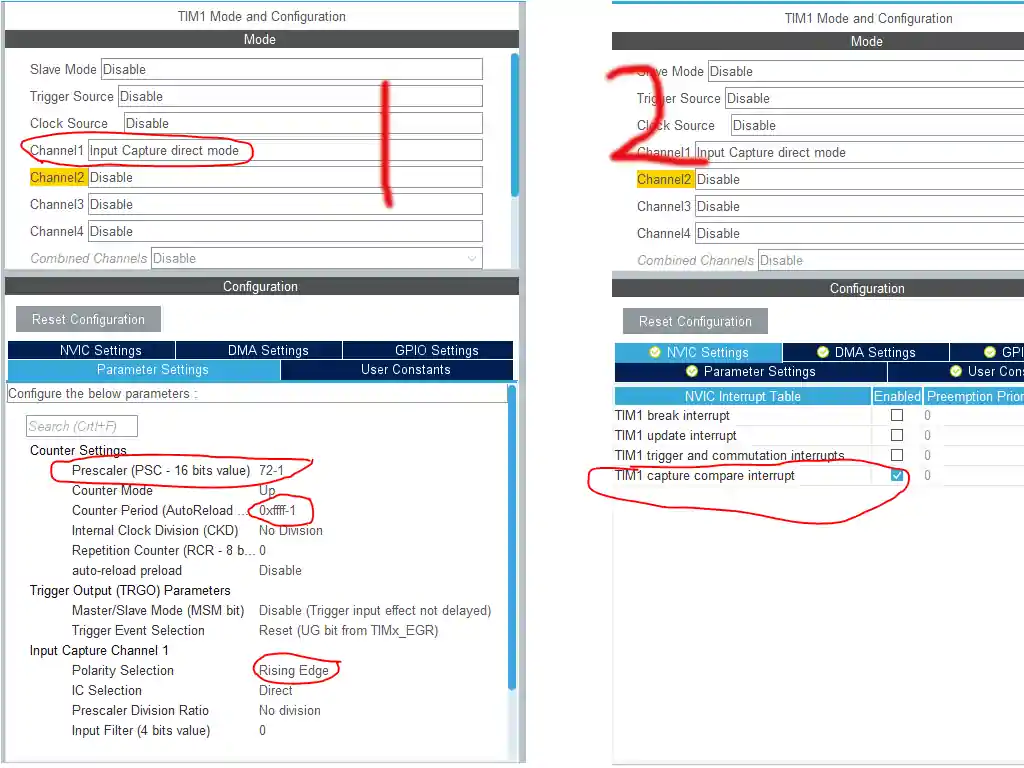HCSR04 timer setup

As shown above, I have selected the Input Capture Direct Mode.
Prescalar is set to 72 -> This will divide the APB clock by 72, and bring the timer clock to 1 MHz. It is necessary because the HCSR04 sends the signal in microseconds.
ARR is set to 0xffff -> I have selected is as maximum as possible. This basically sets the limit, upto which we can count in microseconds.
Also make sure the TIMx Capture Compare interrupt is enabled. You can enable the Global interrupt also, if you don’t see this option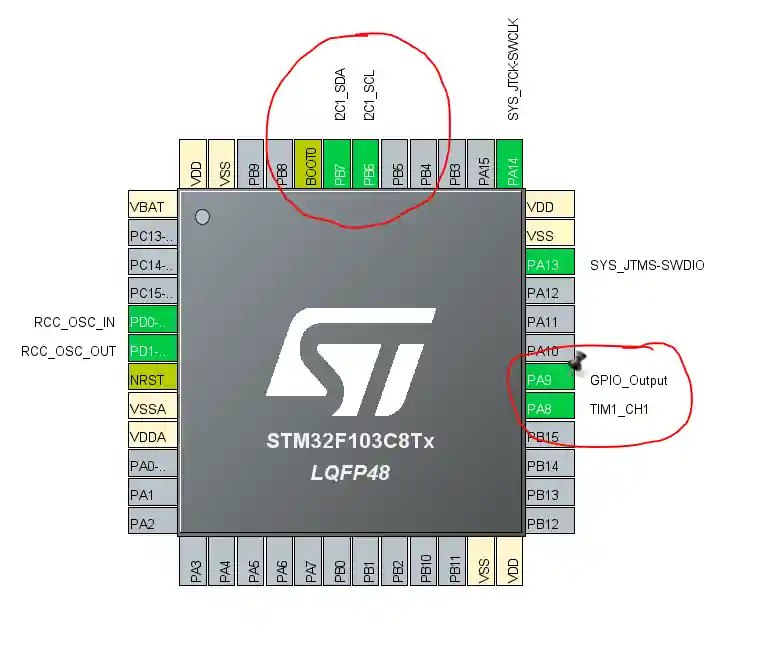HCSR04 pin setup

I2C 1 is selected -> To connect the LCD1602
Pin PA8 -> is automatically selected as TIM1_CH1 pin. I will use it as the ECHO Pin
Pin PA9 -> will be use as TRIG Pin, so select it as the output.

## Connection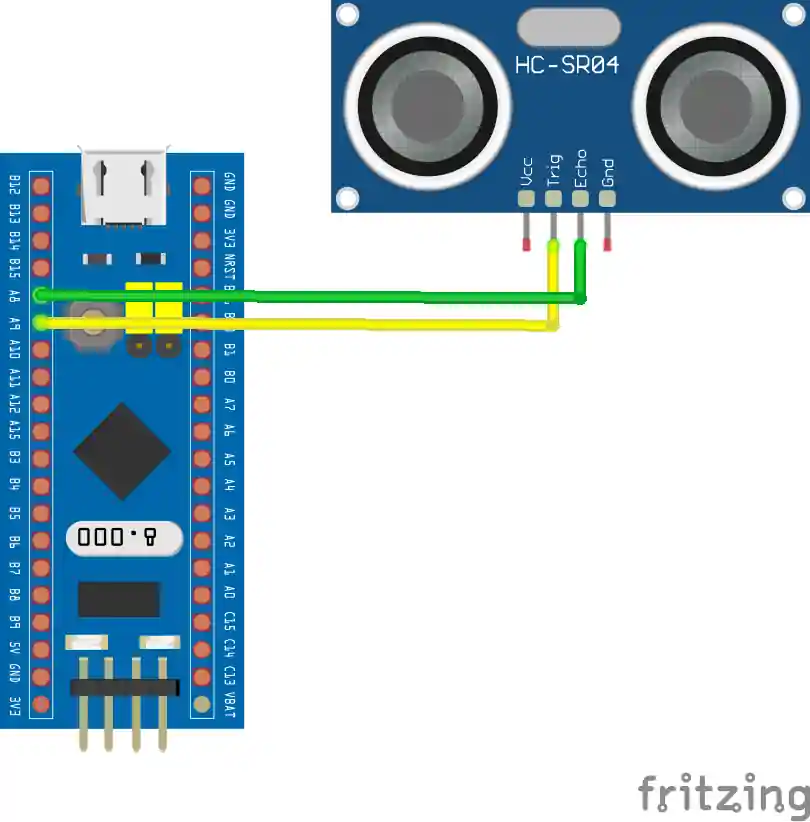HCSR04 Connection

## Some Insight into the CODE

```uint32_t IC_Val1 = 0;
uint32_t IC_Val2 = 0;
uint32_t Difference = 0;
uint8_t Is_First_Captured = 0;  // is the first value captured ?
uint8_t Distance  = 0;

#define TRIG_PIN GPIO_PIN_8
#define TRIG_PORT GPIOA

// Let's write the callback function

void HAL_TIM_IC_CaptureCallback(TIM_HandleTypeDef *htim)
{
if (htim->Channel == HAL_TIM_ACTIVE_CHANNEL_1)  // if the interrupt source is channel1
{
if (Is_First_Captured==0) // if the first value is not captured
{
IC_Val1 = HAL_TIM_ReadCapturedValue(htim, TIM_CHANNEL_1); // read the first value
Is_First_Captured = 1;  // set the first captured as true
// Now change the polarity to falling edge
__HAL_TIM_SET_CAPTUREPOLARITY(htim, TIM_CHANNEL_1, TIM_INPUTCHANNELPOLARITY_FALLING);
}

else if (Is_First_Captured==1)   // if the first is already captured
{
IC_Val2 = HAL_TIM_ReadCapturedValue(htim, TIM_CHANNEL_1);  // read second value
__HAL_TIM_SET_COUNTER(htim, 0);  // reset the counter

if (IC_Val2 > IC_Val1)
{
Difference = IC_Val2-IC_Val1;
}

else if (IC_Val1 > IC_Val2)
{
Difference = (0xffff - IC_Val1) + IC_Val2;
}

Distance = Difference * .034/2;
Is_First_Captured = 0; // set it back to false

// set polarity to rising edge
__HAL_TIM_SET_CAPTUREPOLARITY(htim, TIM_CHANNEL_1, TIM_INPUTCHANNELPOLARITY_RISING);
__HAL_TIM_DISABLE_IT(&htim1, TIM_IT_CC1);
}
}
}
```

In the Function above:-

• First Timestamp is captured, when the rising edge is detected. The polarity is now set for the falling edge
• Second Timestamp will be captured on the falling edge
• Difference between the Timestamps will be calculated. This Difference will be microseconds, as the timer is running at 1 MHz
• Based on the Difference value, the distance is calculated using the formula given in the datasheet
• Finally, the Interrupt will be disabled, so that we don’t capture any unwanted signals.
```void HCSR04_Read (void)
{
HAL_GPIO_WritePin(TRIG_PORT, TRIG_PIN, GPIO_PIN_SET);  // pull the TRIG pin HIGH
delay(10);  // wait for 10 us
HAL_GPIO_WritePin(TRIG_PORT, TRIG_PIN, GPIO_PIN_RESET);  // pull the TRIG pin low

__HAL_TIM_ENABLE_IT(&htim1, TIM_IT_CC1);
}
```

`HCSR04_Read`-> Triggers the sensor to start the measurement.

• It will pull the TRIG Pin HIGH for 10 microseconds, and then pull it LOW.
• This will force the sensor to start the measurement, and the sensor will pull the ECHO Pin HIGH for the respective amount of time
• To measure this time, we will enable the Timer interrupt, so that we can capture this Rising and falling edges

## RESULT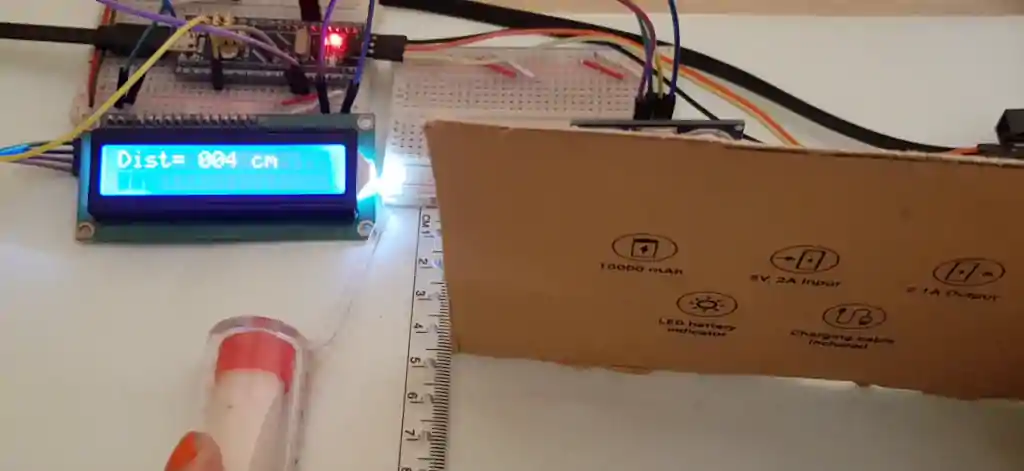hcsr04 working
Check out the VIDEO Below

###### 100%
100%
Subscribe
Notify of28 Comments
Inline Feedbacks
View all comments
Menu